# Merger Model

Guide to Understanding Accretion / (Dilution) Analysis in Merger Models## Merger Model Overview

The mergers and acquisitions (M&A) group in investment banking provides advisory services on either sell-side or buy-side transactions.

• Sell-Side M&A → The client advised by the bankers is the company (or owner of the company) is seeking a partial or complete sale.
• Buy-Side M&A → The client advised by the bankers is the buyer interested in purchasing a company or part of a company, such as acquiring the division of a company undergoing a divestiture.

But irrespective of which side the represented client is on, the proper understanding of the mechanics of building a merger model is a critical part of the job.

Specifically, the underlying purpose of the merger model is to perform accretion / (dilution) analysis, which determines the anticipated impact of an acquisition on the earnings per share (EPS) of the acquirer upon transaction-close.

In M&A transactions, “accretion” refers to an increase in the pro forma EPS post-deal, whereas “dilution” indicates a decline in EPS after transaction-close.

## How to Build a Merger Model

The process of building a merger model consists of the following steps:

• Step 1: Determine the Offer Value Per Share (and Total Offer Value)
• Step 2: Structure the Purchase Consideration (i.e. Cash, Stock, or Mix)
• Step 3: Estimate the Financing Fee, Interest Expense, Number of New Share Issuances, Synergies, and Transaction Fee
• Step 4: Perform Purchase Price Accounting (PPA), i.e. Calculate Goodwill and Incremental D&A
• Step 5: Calculate the Standalone Earnings Before Taxes (EBT)
• Step 6: Move from the Consolidated EBT to the Pro Forma Net Income
• Step 7: Divide the Pro Forma Net Income by the Pro Forma Diluted Shares Outstanding to Arrive at the Pro Forma EPS
• Step 8: Estimate the Accretive (or Dilutive) Impact on the Pro Forma EPS

For the final step, the following formula calculates the net EPS impact.

###### EPS Accretion / (Dilution) Formula
• Accretion / (Dilution) = (Pro Forma EPS / Standalone EPS) – 1

## Importance of Accretion / (Dilution) Analysis in M&A

So why do companies pay such close attention to the post-deal EPS?

Generally, the analysis pertains mainly to publicly-traded companies because their market valuations are often on the basis of their earnings (and EPS).

• Accretive → Increase in Pro Forma EPS
• Dilutive → Decrease in Pro Forma EPS

With that said, a decline in EPS (i.e. “dilution”) tends to be perceived negatively and signals the acquirer might have overpaid for an acquisition – in contrast, the market views an increase in EPS (i.e. “accretion”) positively.

Of course, accretion/dilution analysis cannot determine whether an acquisition will actually pay off or not.

However, the model still ensures that the market’s reaction to the deal’s announcement (and potential impact on the share price) is taken into account.

For example, let’s say an acquirer’s standalone EPS is \$1.00, but it increases to \$1.10 post-acquisition.

The market will likely interpret the acquisition positively, rewarding the company with a higher share price (and the reverse would be true for a reduction in EPS).

• EPS Accretion / (Dilution) = (\$1.10 / \$1.00) – 1 = 10%

The proposed M&A transaction is thereby 10% accretive, so we’re forecasting a \$0.10 increase in EPS.

## Merger Model Tutorial — Excel Template

We’ll now move to a modeling exercise, which you can access by filling out the form below.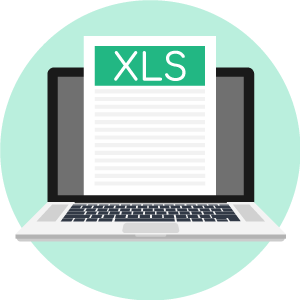Submitting ...

## Acquirer and Target Financial Profile

Suppose an acquirer is amidst pursuing an acquisition of a smaller-sized target company, and you’re tasked with building a merger model to advise on the transaction.

On the date of the analysis, the acquirer’s share price is \$40.00 with 600 million diluted shares outstanding – therefore, the acquirer’s equity value is \$24 billion.

• Share Price = \$40.00
• Diluted Shares Outstanding = 600 million
• Equity Value = \$40.00 * 600 million = \$24 billion

If we assume the acquirer’s forecasted earnings per share (EPS) is \$4.00, the implied P/E multiple is 10.0x.

As for the financial profile of the target, the company’s latest share price was \$16.00 with 200 million shares outstanding, meaning the equity value is \$3.2 billion.

• Share Price = \$16.00
• Diluted Shares Outstanding = 200 million
• Equity Value = \$16.00 * 200 million = \$3.2 billion

The target’s forecasted EPS is assumed to be \$2.00, so the P/E ratio is 8.0x, which is 2.0x turns lower than the acquirer’s P/E.

In order to acquire the target, the acquirer must bid an offer price per share with a sufficient premium to incentivize the company’s board and shareholders to accept the proposal.

Here, we’ll assume the offer premium is 25.0% over the target’s current share price of \$16.00, or \$20.00.

• Offer Price Per Share = \$16.00 * (1 + 25%) = \$20.00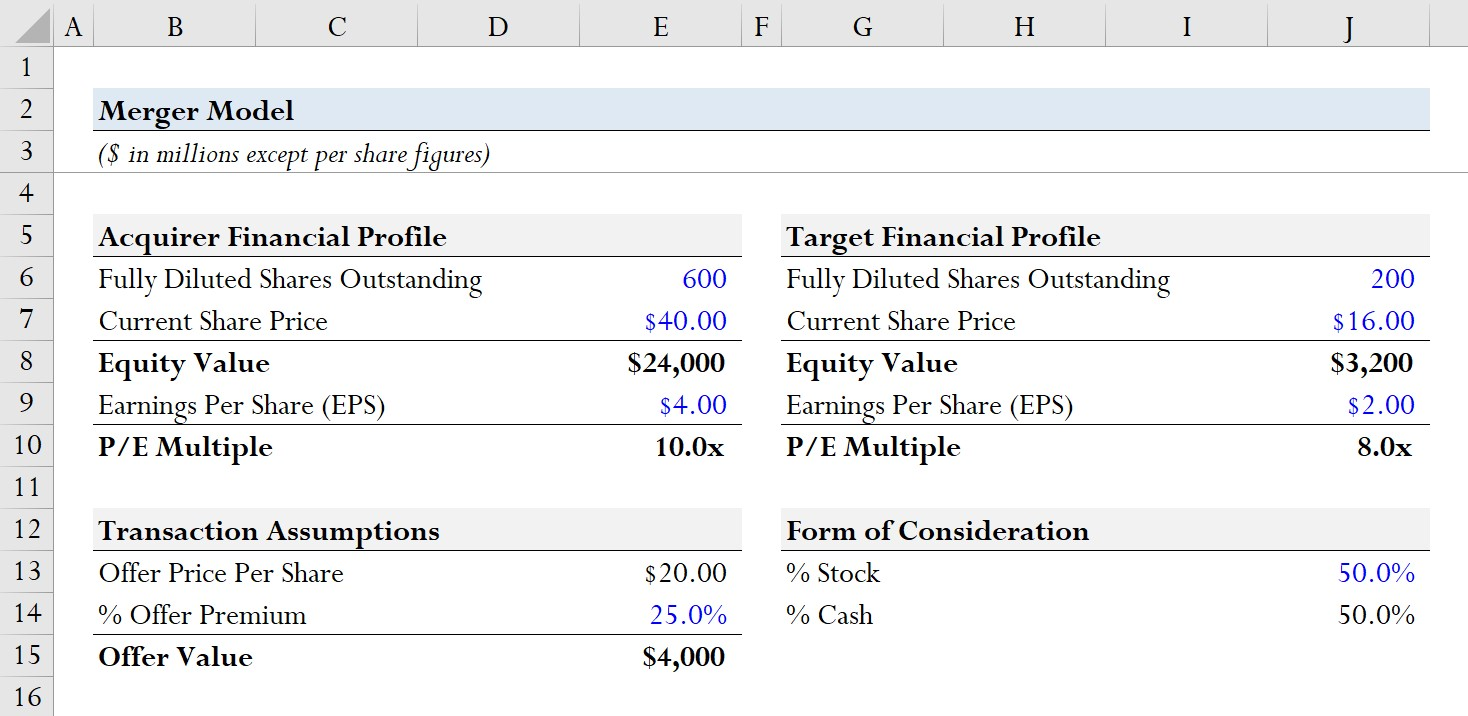## Transaction Assumptions – Cash vs. Stock Consideration

Since the target’s diluted share count is 200 million, we can multiply that amount by the \$20.00 offer price per share for an estimated offer value of \$4 billion.

• Offer Value = \$20.00 * 200 million = \$4 billion

With regards to the acquisition financing – i.e. the forms of consideration – the deal is funded 50.0% using stock and 50.0% using cash, with the cash component stemming entirely from newly raised debt.

• % Cash Consideration = 50.0%
• % Stock Consideration = 50.0%

Given the offer value of \$4 billion and 50% cash consideration, \$2 billion in debt was used to fund the purchase, i.e. the cash consideration side.

If we assume the financing fees incurred in the process of raising the debt capital were 2.0% of the total debt amount, the total financing fee is assumed to be \$40 million.

• Total Financing Fee = \$2 billion * 2.0% = \$40 million

The accounting treatment of financing fees is akin to that of depreciation and amortization, where the fee is allocated across the borrowing term.

Assuming a borrowing term of 5 years, the financing fee amortization is \$8 million per year for the next five years.

• Financing Fee Amortization = \$40 million / 5 Years = \$8 million

Since interest must be paid on the new debt raised to fund the purchase, we’ll assume the interest rate is 5.0% and multiply that rate by the total debt to calculate annual interest expense of \$100 million.

• Annual Interest Expense = 5.0% * \$2 billion = \$100 million

With the cash consideration section complete, we’ll move to the stock consideration side of the deal structure.

Like our calculation of total debt, we’ll multiply the offer value by the % stock consideration (50%) to arrive at \$2 billion.

The number of new acquirer shares issued is calculated by dividing the stock consideration by the current share price of the acquirer, \$40.00, which implies that 50 million new shares must be issued.

• Number of Acquirer Shares Issued = \$2 billion / \$40.00 = 50 million

In the next section, we must address two more assumptions:

1. Synergies
2. Transaction Fees

Synergies represent the incremental revenue generation or cost savings from the transaction, which we’ll net against the costs of the synergies, i.e. the losses from the integration process and shutting down facilities, even if those represent cost savings over the long run.

The synergies from our hypothetical deal are assumed to be \$200 million.

• Synergies, net = \$200 million

The transaction fees – i.e. the expenses related to M&A advisory to investment banks and lawyers – are assumed to be 2.5% of the offer value, which comes out to be \$100 million.

• Transaction Fees = 2.5% * \$4 billion = \$100 million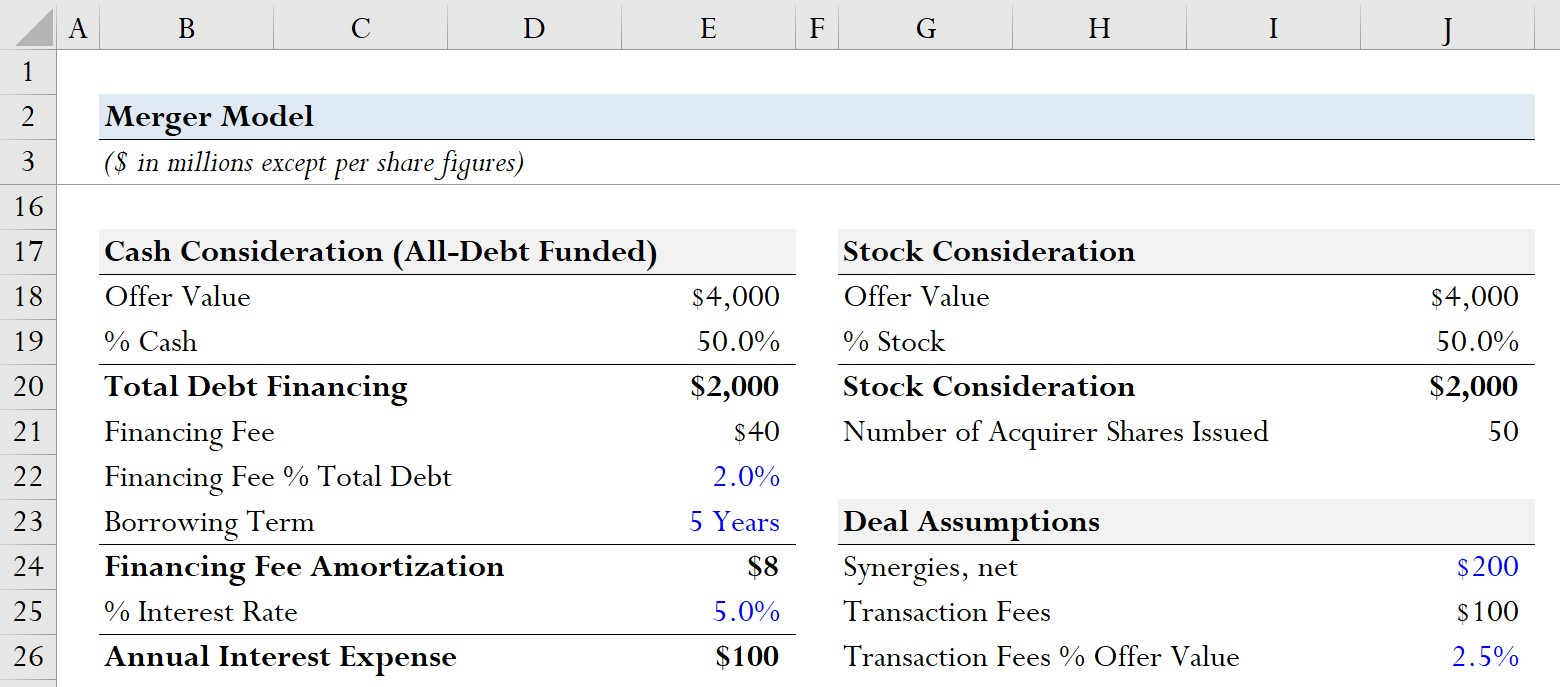## Purchase Price Accounting (PPA)

In purchase price accounting, the newly acquired assets are re-evaluated and adjusted to their fair value, if deemed appropriate.

The purchase premium is the excess of the offer value over the net tangible book value, which we’ll assume is \$2 billion.

The purchase premium is allocatable to the write-up of PP&E and intangibles, with the remaining amount recognized as goodwill, an accrual accounting concept meant to capture the “excess” paid over the fair value of a target’s assets.

The allocation of the purchase premium is 25% to PP&E and 10% to intangibles, with both having a useful life assumption of 20 years.

• PP&E Write-Up
• % Allocation to PP&E = 25.0%
• PP&E Useful Life Assumption = 20 Years
• Intangibles Write-Up
• % Allocation to Intangibles = 10.0%
• Intangibles Useful Life Assumption = 20 Years

Upon multiplying the purchase premium by the allocation percentages, the PP&E write-up is \$500 million, while the intangibles write-up is \$200 million.

• PP&E Write-Up = 25% * \$2 billion = \$500 million
• Intangibles Write-Up = 10% * \$2 billion = \$200 million

However, the write-up of PP&E and intangibles creates a deferred tax liability (DTL), which arises from a temporary timing difference between GAAP book taxes and the cash taxes paid to the IRS.

Since future cash taxes will exceed the book taxes shown on the financial statements, a DTL is recorded on the balance sheet to offset the temporary tax discrepancy that will gradually decline to zero.

The incremental depreciation stemming from the PP&E and intangibles write-ups are tax-deductible for book purposes but not deductible for tax purposes.

The incremental depreciation is \$25 million, while the incremental amortization is \$10 million.

• Incremental Depreciation = \$500 million / 20 Years = \$25 million
• Incremental Amortization = \$200 million / 20 Years = \$10 million
• Incremental D&A = \$25 million + \$10 million = \$35 million

The annual decline of the DTLs will be equal to the total DTL divided by the corresponding useful life assumptions for each asset that is written up.

The total goodwill created is \$1.4 billion, which we calculated by subtracting the write-ups from the purchase premium and adding the DTL.

• Goodwill Created = \$2 billion – \$500 million – \$200 million + \$140 million
• Goodwill = \$1.4 billion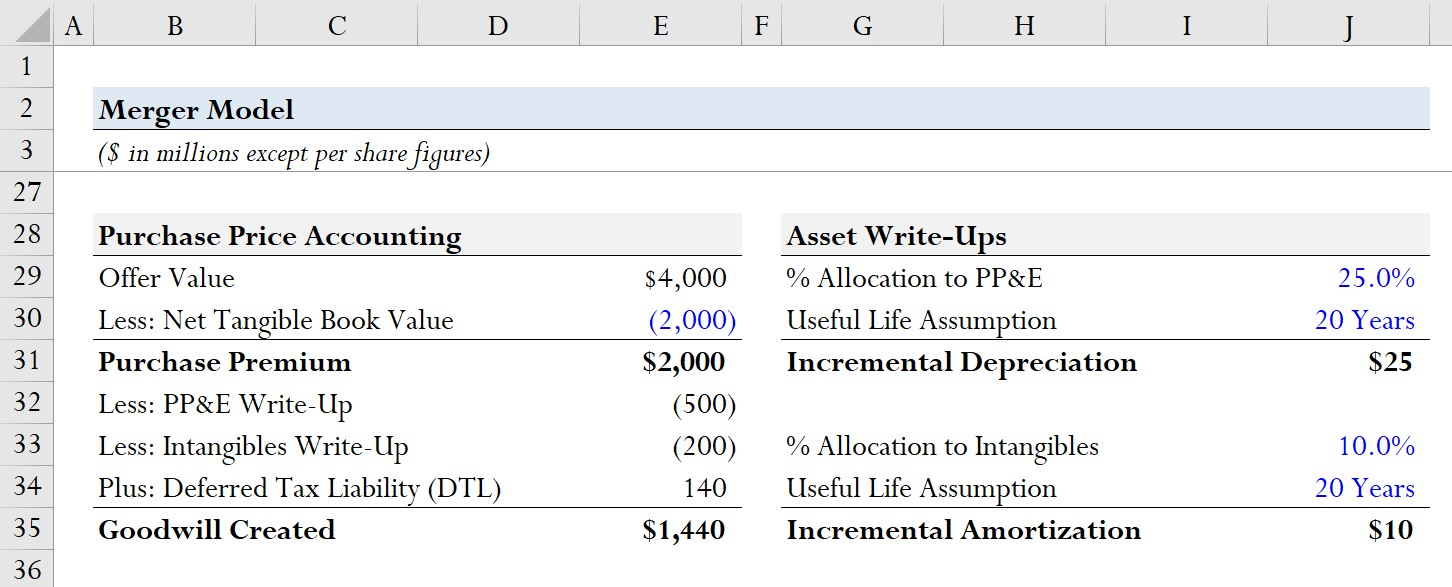## Accretion/Dilution Analysis

In the final part of our exercise, we’ll start by calculating each company’s net income and earnings before taxes (EBT) on a standalone basis.

The net income can be calculated by multiplying the forecasted EPS by the diluted shares outstanding.

• Acquirer Net Income = \$4.00 * 600 = \$2.4 billion
• Target Net Income = \$2.00 * 200 = \$4 million

EBT can be calculated by dividing the company’s net income by one minus the tax rate, which we’ll assume to be 20.0%.

• Acquirer EBT = \$2.4 billion / (1 – 20%) = \$3 billion
• Target EBT = \$400 million / (1 – 20%) = \$500 million

We’ll now work to determine the post-acquisition company’s pro forma combined financials.

• Consolidated EBT = \$3 billion + \$500 million = \$3.5 billion
• Less: Interest Expense and Financing Fees Amortization = \$108 million
• Less: Transaction Fees = \$100 million
• Plus: Synergies, net = \$200 million
• Less: Incremental Depreciation = \$35 million
• Pro Forma Adjusted EBT = \$3.5 billion

From there, we must deduct taxes using our 20% tax rate assumption.

• Taxes = \$3.5 billion * 20% = \$691 million

The pro forma net income is \$2.8 billion, which is the numerator in our EPS calculation.

• Pro Forma Net Income = \$3.5 billion – \$691 million = \$2.8 billion

Before the transaction, the acquirer had 600 million diluted shares outstanding, but to partially finance the deal, 50 million more shares were newly issued.

• Pro Forma Diluted Shares = 600 million + 50 million = 650 million

By dividing our pro forma net income by our pro forma share count, we arrive at a pro forma EPS for the acquirer of \$4.25.

• Pro Forma EPS = \$2.8 billion / 650 million = \$4.25

Compared to the initial pre-deal EPS of \$4.00, that represents an increase in EPS of \$0.25.

In closing, the implied accretion / (dilution) is 6.4%, which was derived by dividing the pro forma EPS by the pre-deal EPS of the acquirer, and then subtracting 1.

• % Accretion / (Dilution) = \$4.25 / \$4.00 – 1 = 6.4%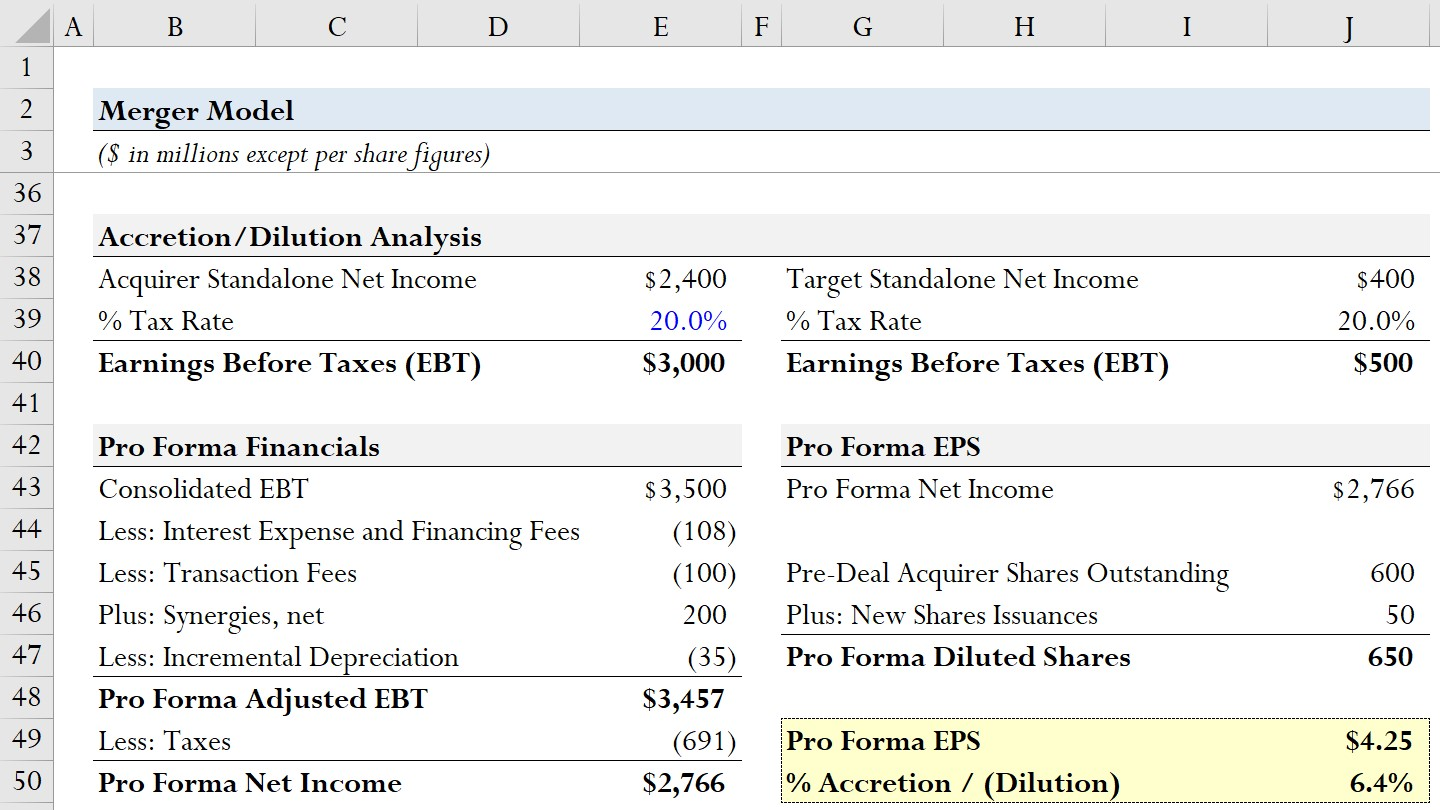Step-by-Step Online Course

#### Everything You Need To Master Financial Modeling

Enroll in The Premium Package: Learn Financial Statement Modeling, DCF, M&A, LBO and Comps. The same training program used at top investment banks.Inline FeedbacksLearn Financial Modeling Online

Everything you need to master financial and valuation modeling: 3-Statement Modeling, DCF, Comps, M&A and LBO.

X

The Wall Street Prep Quicklesson Series

7 Free Financial Modeling Lessons

Get instant access to video lessons taught by experienced investment bankers. Learn financial statement modeling, DCF, M&A, LBO, Comps and Excel shortcuts.